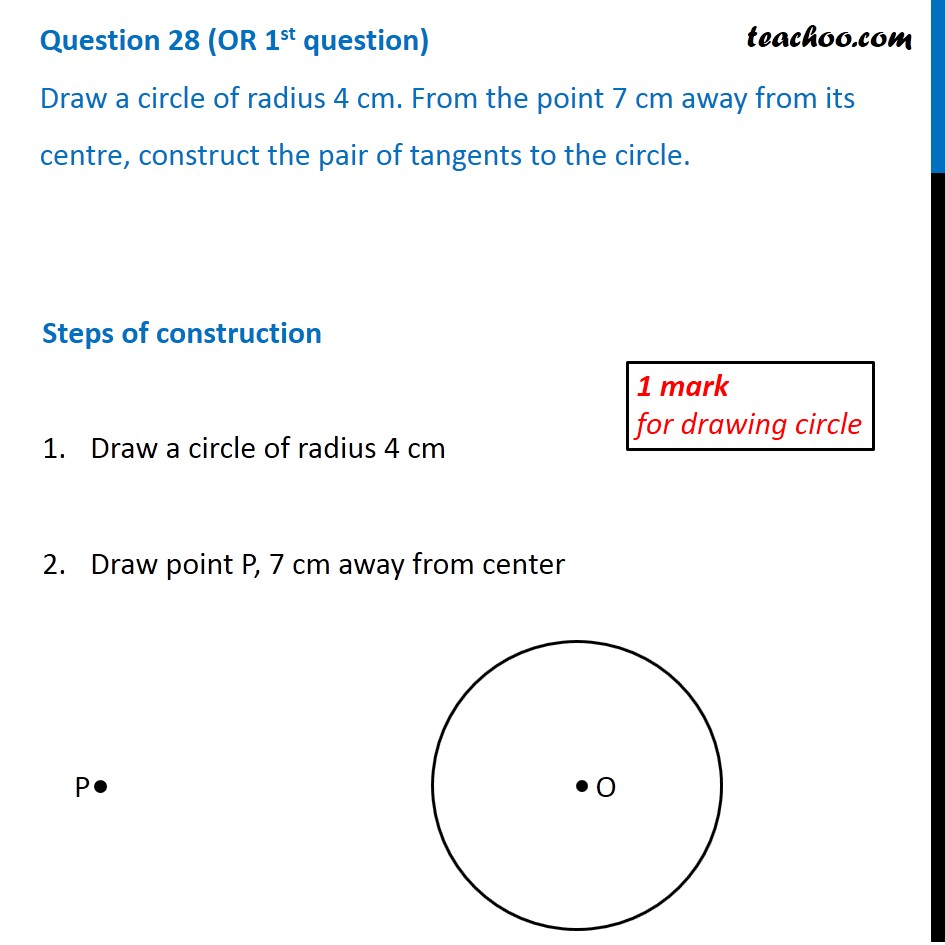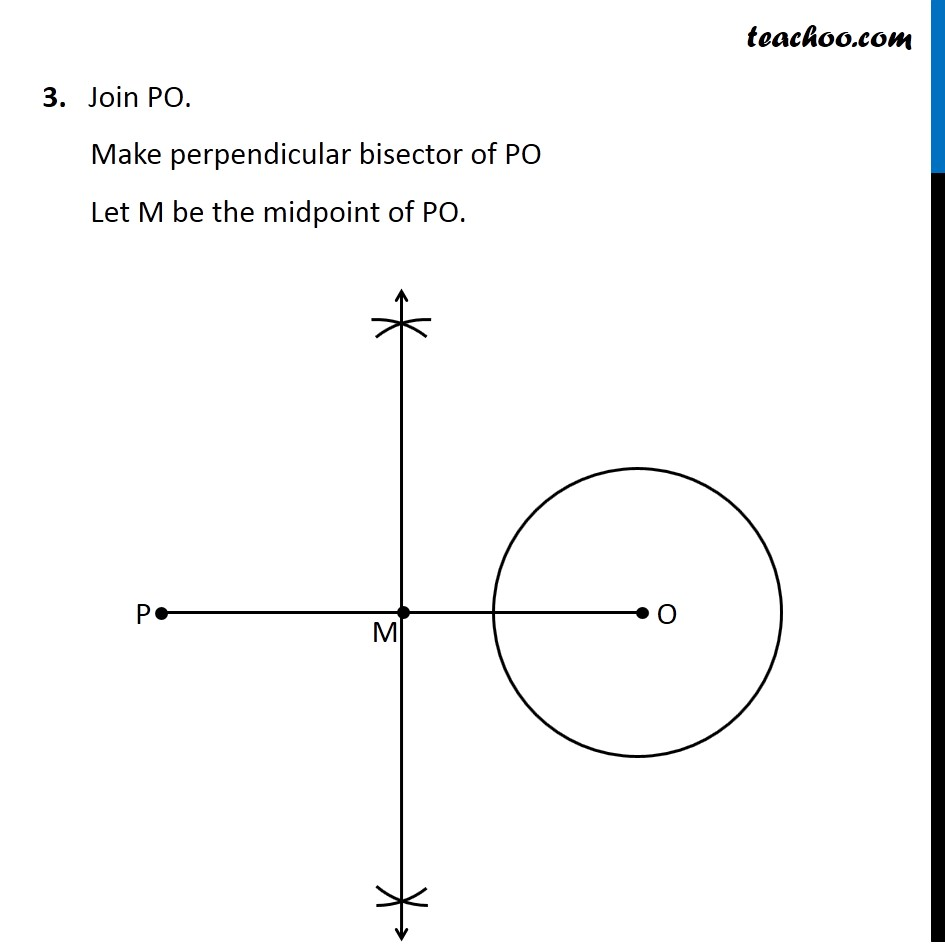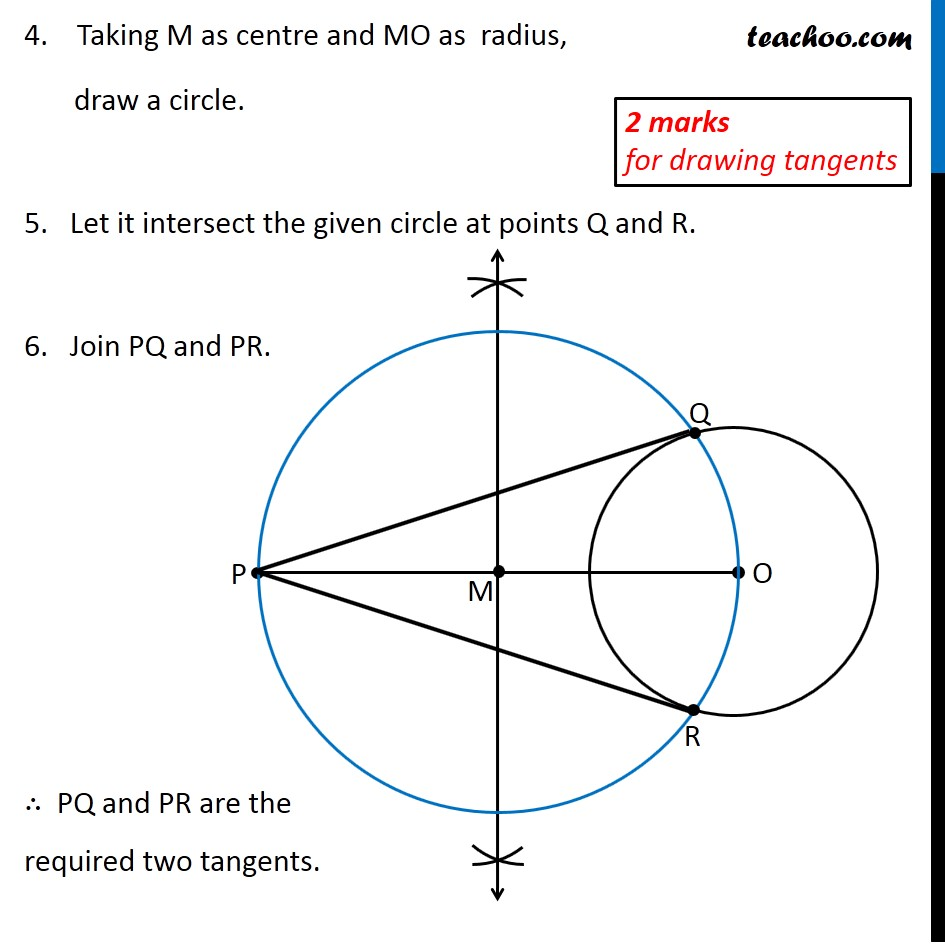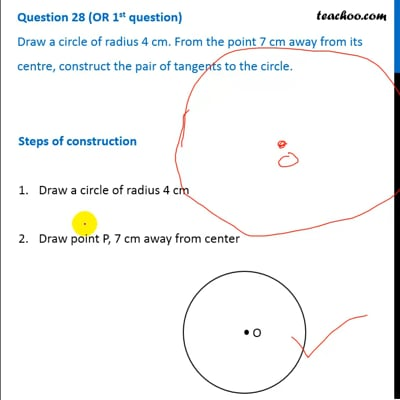CBSE Class 10 Sample Paper for 2020 Boards - Maths Basic

Class 10
Solutions of Sample Papers for Class 10 Boards

## Draw a circle of radius 4 cm. From the point 7 cm away from its centre, construct the pair of tangents to the circle.This video is only available for Teachoo black users

Note : This is similar to Ex 11.2, 1 of NCERT – Chapter 11 Class 10

### Transcript

Question 28 (OR 1st question) Draw a circle of radius 4 cm. From the point 7 cm away from its centre, construct the pair of tangents to the circle. Steps of construction Draw a circle of radius 4 cm Draw point P, 7 cm away from center 1 mark for drawing circle 3. Join PO. Make perpendicular bisector of PO Let M be the midpoint of PO. 4. Taking M as centre and MO as radius, draw a circle. 5. Let it intersect the given circle at points Q and R. 6. Join PQ and PR. ∴ PQ and PR are the required two tangents.